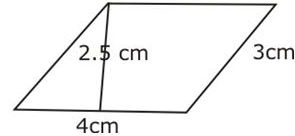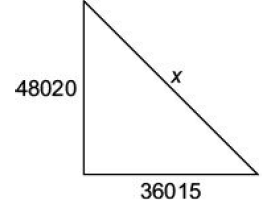Courses

# Test: Mensuration- 4

## 15 Questions MCQ Test Quantitative Aptitude for SSC CGL | Test: Mensuration- 4

Description
This mock test of Test: Mensuration- 4 for Quant helps you for every Quant entrance exam. This contains 15 Multiple Choice Questions for Quant Test: Mensuration- 4 (mcq) to study with solutions a complete question bank. The solved questions answers in this Test: Mensuration- 4 quiz give you a good mix of easy questions and tough questions. Quant students definitely take this Test: Mensuration- 4 exercise for a better result in the exam. You can find other Test: Mensuration- 4 extra questions, long questions & short questions for Quant on EduRev as well by searching above.
QUESTION: 1

### Find the volume of a cuboid whose length is 8 cm, breadth 6 cm and height 3.5 cm.

Solution:

Explanation:

Length of the cuboid = 8 cm

Breadth of the cuboid = 6 cm

Height of the cuboid = 3.5 cm

Volume of the cuboid = length × breadth × height

= 8 x 6 x 3.5 = 168cm3

Therefore,volume of  the cuboid = 168cm3

QUESTION: 2

### Find the area of the given figure.Solution:

Explanation:

Area of  a parallellogram =  Base x Height = 4 x 2.5 = 10 cm2

QUESTION: 3

### Find the volume of a cuboid whose length is 8 cm, width is 3 cm and height is 5 cm.

Solution:

Explanation:Length of  the cuboid = 8 cmWidth of the cuboid = 3 cmHeight of the cuboid = 5 cmVolume of  a cuboid  = length × breadth × heightTherefore, Volume of the given  cuboid = 8x3x5 = 120 cm3

QUESTION: 4

Find the perimeter of a rectangle whose length is 4 cm and breadth is 3 cm.

Solution:

Explanation:Length of the rectangle,l = 4cmBreadth of the rectangle,b = 3 cmPerimeter of a rectangle = 2(l+b) = 2(4+3) = 2x7 = 14 cm

QUESTION: 5

Area of a rhombus = ______

Solution:

Explanation:Area of a rhombus = Half the product of its diagonals                               = 1/2 x d1x d2

QUESTION: 6

Area of a trapezium = Half of the sum of the lengths of parallel sides × ______

Solution:

Explanation:Area of a trapezium =( Half of the sum of the length of parallel sides )×(perpendicular distance between them)

QUESTION: 7

Surface area of a cuboid = __________

Solution:

Explanation:Surface area of a cuboid = 2(lb + bh + hl)where, l= lengthb = breadthand h = height

QUESTION: 8

_________ of a solid is the sum of the areas of its faces.

Solution:

Explanation:The surface area of a three-dimensional figure is the sum of the areas of all its faces.

QUESTION: 9

In a right angled triangle, find the hypotenuse if base and perpendicular are respectively 36015 cmand 48020 cm.

Solution:Let hypotenuse = x cm
Then, by Pythagoras theorem:
x2 = (48020)2 + (36015)2
x fi 60025 cm

QUESTION: 10

The inner circumference of a circular track is 440 cm. The track is 14 cm wide. Find the diameterof the outer circle of the track.

Solution:

Let inner radius = A; then 2pr = 440 \ p = 70
Radius of outer circle = 70 + 14 = 84 cm
Outer diameter = 2 × Radius = 2 × 84 = 168

QUESTION: 11

A pit 7.5 metre long, 6 metre wide and 1.5 metre deep is dug in a field. Find the volume of soilremoved in cubic metres.

Solution:

Volume of soil removed = l × b × h
= 7.5 × 6 × 1.5 = 67.5 m3

QUESTION: 12

The whole surface of a rectangular block is 8788 square cm. If length, breadth and height are inthe ratio of 4 : 3 : 2, find length.

Solution:

Let the common ratio be = x
Then, length = 4x, breadth = 3x and height = 2x
As per question;
2(4x x 3x + 3x x 2x + 2x x 4x) = 8788
2(12x2 + 6x2 + 8x2) = 8788 fi 52x2 = 8788
fi x = 13
Length = 4x = 52 cm

QUESTION: 13

Find the height of a Cuboid , if the volume and area of its base is 1240cm3 and 40 sq.cm respectively.

Solution:
QUESTION: 14

A bicycle wheel makes 5000 revolutions in moving 11 km. What is the radius of the wheel?

Solution:

Let the radius of the wheel be = p
Then 5000 × 2pr = 1100000 cm fi r = 35 cm

QUESTION: 15

The short and the long hands of a clock are 4 cm and 6 cm long respectively. What will be sum ofdistances travelled by their tips in 4 days? (Take p = 3.14)

Solution:

In 4 days, the short hand covers its circumference
4 × 2 = 8 times long hand covers its circumference
4 × 24 = 96 times
Then they will cover a total distance of:-
(2 × p × 4)8 + (2 × p × 6)96 fi 3818.24 cm